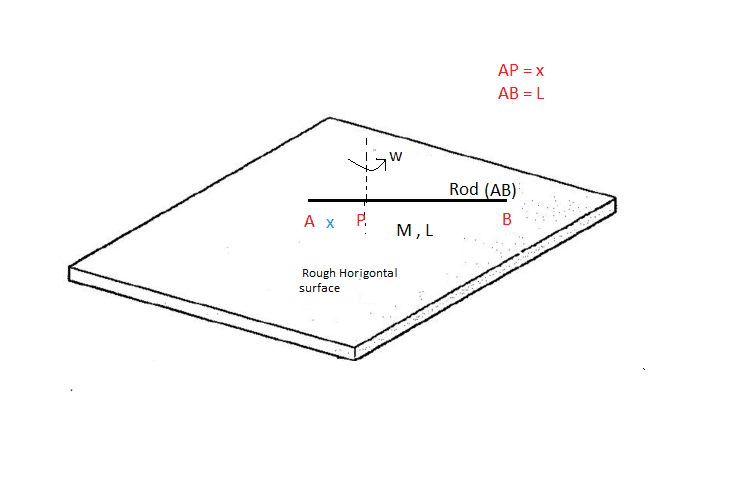# Maxima and Minima, Rod Rotating on a Surface With Friction

• Phys student

## Homework StatementA uniform rod of mass M and length L can rotate around point P which is at position x from one end of the rod as shown in the figure. The rod is gently placed on a rough horizontal surface that has a friction coefficient μ. and at t=0 starts rotating with angular velocity ω.

The total time of rotation of the rod is T, which depends on x. Let the maximum and minimum time of rotation be T_M and T_m respectively. Find: $$\frac{T_m}{T_M}$$

## The Attempt at a Solution

We need to find the torque that the friction force exerts on the rod and from that find the angular deceleration of the rod. We need to consider the part of the rod that is above the point of rotation and the one below it. Let's take a small mass dm with length ds, we have (λ is the linear mass density of the rod) :

$$dF_f = μgdm = μgλds$$
$$dτ= μgλsds$$
The total torque is the sum of the torques of the two parts:
$$\int_{0}^{L-x} μgλs ds + \int_{0}^{-x} μgλs ds = \frac{1}{2}μgλx^2 + \frac{1}{2}μgλ(L-x)^2 = \frac{1}{2}μgλ(L^2 +2(x^2-Lx))$$
Now:
$$α=\frac{τ}{I}= \frac{\frac{1}{2}μgλ(L^2 +2(x^2-Lx))}{\frac{1}{3}ML^2 +Mx^2}= \frac{μgλ(L^2 +2(x^2-Lx))}{\frac{2}{3}ML^2 +2Mx^2}$$
$$T= \frac{ω}{α} = \frac{ω(\frac{2}{3}ML^2 +2Mx^2)}{μgλ(L^2 +2(x^2-Lx))}$$

We take the derivative with respect to x and set it equal to 0:
$$\frac{d}{dx} \frac{ω(\frac{2}{3}ML^2 +2Mx^2)}{μgλ(L^2 +2(x^2-Lx))} = \frac{4LMω(L^2+Lx-3x^2)}{3gλμ(L^2-2Lx+2x^2)} = \frac{4L^2ω(L^2+Lx-3x^2)}{3gμ(L^2-2Lx+2x^2)} = 0$$
When we solve for x we get one negative root which isn't physical.
$$x= \frac{-L+L\sqrt{13}}{-6} = \frac{L(1-\sqrt{13})}{6}$$
Can someone please tell me where's the mistake?

Last edited:

$$\frac{\frac{1}{2}μgλ(L^2 +2(x^2-Lx))}{\frac{1}{3}ML^2 +Mx^2}$$
How do you get that expression for I?

•Phys student
How do you get that expression for I?

I used the parallel axis theorem. But I think I made a mistake, because the theorem applies to the moment of inertia around the center of mass so it should have been:
$$I=\frac{1}{12}ML^2 +M(\frac{L}{2} -x)^2$$

Last edited:
Oh, The parallel axis theorem applies to the moment of inertia around the center of mass so it should have been:
$$I=\frac{1}{12}ML^2 +M(\frac{L}{2} -x)^2$$
Yes. Does the answer look better now?

•Phys student
Yes. Does the answer look better now?

We have:
$$α=\frac{μgλ(L^2 +2(x^2-Lx))}{\frac{1}{6}ML^2 +2M(\frac{L}{2} -x)^2}$$
$$T= \frac{ω}{α} = \frac{ω(\frac{1}{6}ML^2 +2M(\frac{L}{2} -x)^2)}{μgλ(L^2 +2(x^2-Lx))}$$
When we take the derivative we get:
$$\frac{d}{dx} \frac{ω(\frac{1}{6}ML^2 +2M(\frac{L}{2} -x)^2)}{μgλ(L^2 +2(x^2-Lx))} = -\frac{2L^2Mω(L-2x)}{3μgλ(L^2-2Lx+2x^2)^2}$$
Which only gives us one solution: $$x= \frac{L}{2}$$
We should have two solutions. So, there's still something wrong

Last edited:
##
\frac{d}{dx} \frac{ω(\frac{1}{6}ML^2 +2M(\frac{L}{2} -x)^2)}{μgλ(L^2 +2(x^2-Lx))} = -\frac{2L^2Mω(L-2x)}{3μgλ(L^2-2Lx+2x^2)^2}##
That's not what I get.
Which only gives us one solution:
It is possible there is only one local extremum. There is a physical constraint on the range of x.

•Phys student
That's not what I get.

It is possible there is only one local extremum. There is a physical constraint on the range of x.

I guess it could be possible that there's only one extremum but it would mean that we don't have both a maximum and minimum time.

Last edited:
I guess it could be possible that there's only one extremum but it would mean that we don't have both a maximum and minimum time.
No, I wrote local extremum, but even that wasn't quite accurate. One end of the physical range for x may be an extremum by virtue of the fact that x cannot go any further along, but the gradient need not be zero. Indeed, the fact that there was only one d/dx = 0 point, and that the two ends of the physical range are equivalent, it follows that those end points must represent the other extreme value.

•Phys student
No, I wrote local extremum, but even that wasn't quite accurate. One end of the physical range for x may be an extremum by virtue of the fact that x cannot go any further along, but the gradient need not be zero. Indeed, the fact that there was only one d/dx = 0 point, and that the two ends of the physical range are equivalent, it follows that those end points must represent the other extreme value.
I see what you mean now.

If we substitute $x= \frac{L}{2}$ we get:

$$T_m = \frac{Mω}{3gλμ}$$

Now, when we substitute $x=0$ or $x=L$ we get:
$$T_M = \frac{2Mω}{3gλμ}$$

So:
$$\frac{T_m}{T_M} = \frac{1}{2}$$

Can this result be derived intuitively, without calculus?

Anyway, thank you very much for you help.

Can this result be derived intuitively, without calculus?
Perhaps.
A rod length L pivoted at one end will clearly take the same time to stop as two rods of length L, and therefore the same as a single rod length 2L pivoted about its centre.
Doubling the length of the rod (for the same density) doubles the mass, multiplies the moment of inertia by 8, so multiplies the KE by 8. The frictional force doubles, so the rotational distance to stop quadruples. Under uniform acceleration, four time the distance means twice the time.
Of course, I haven't shown these are the extreme times.

•Phys student